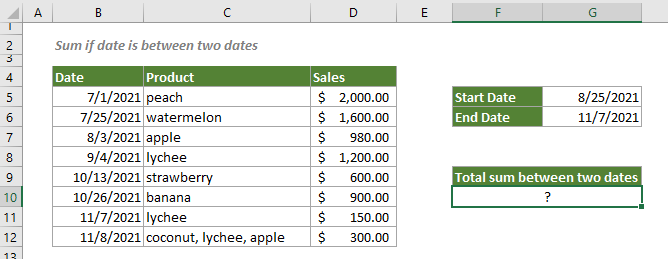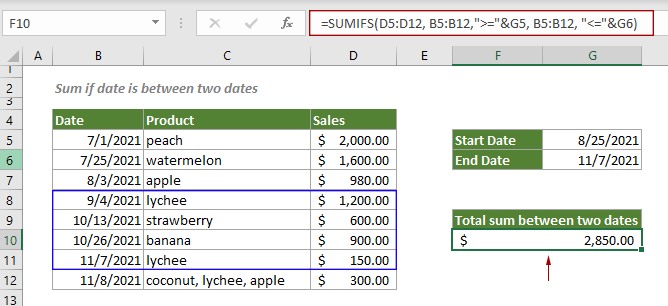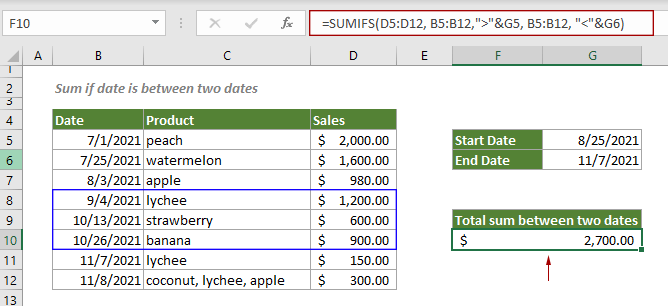## Sum if date is between two dates in Excel

To sum values within a certain date range in Excel, you can apply a formula based on the SUMIFS function.

#### How to sum values that are between two dates in Excel?

This example is going to sum values in column D if the dates in column B are between two given dates provided in G5 and G6.Generic Formulas

Including given dates

=SUMIFS(sum_range, date_range,">="&start_date, date_range, "<="&end_date)

Excluding given dates

SUMIFS(sum_range, date_range,">"&start_date, date_range, "<"&end_date)

Arguments

Sum_range: The range of cells to sum;
Date_range: The range of date cells that you want evaluate by the start_date and end_date;
Start_date: The start date of the date range that defines which cells will be summed;
End_date: The end date of the date range that defines which cells will be summed.

How to use this formula?

Select a blank cell, copy or enter the formula below and press the Enter key to get the result.

Sum values that are between two dates (including these two dates)

=SUMIFS(D5:D12, B5:B12,">="&G5, B5:B12, "<="&G6)Sum values that are between two dates (excluding these two dates)

SUMIFS(D5:D12, B5:B12,">"&G5, B5:B12, "<"&G6)Note: In the formulas, you can change the cells containing the start date and end date, or directly type the start date and end date enclosed in double quotation marks.

#### Related functions

Excel SUMIFS function
The Excel SUMIF function can help to sum cell values based on multiple criteria.

#### Related formulas

Sum if cells contain an asterisk
To sum cells containing an asterisk that is regarded as a literal character not a wildcard, this tutorial explains a formula based on the SUMIF function and the tilde (~).

Sum if cells contain both x and y
This step-by-step tutorial demonstrates how to sum a range of cells if the corresponding cells containing both x and y by using the SUMIFS function.

Sum if cells contain or equal to either x or y
To sum the cells if contain or equal to either one value or another, this tutorial provides two formulas in detail to help solve the problems.

Sum if cells are equal or not equal to a certain value
This step-by-step tutorial explains two formulas to sum a range of cells that equal or not euqal to a certain value based on the SUMIF function.

### The Best Office Productivity Tools

#### Kutools for Excel - Helps You To Stand Out From Crowd

 Popular Features: Find, Highlight or Identify Duplicates  |  Delete Blank Rows  |  Combine Columns or Cells without Losing Data  |  Round without Formula ... Super VLookup: Multiple Criteria  |  Multiple Value  |  Across Multi-Sheets  |  Fuzzy Lookup... Adv. Drop-down List: Easy Drop Down List  |  Dependent Drop Down List  |  Multi-select Drop Down List... Column Manager: Add a Specific Number of Columns  |  Move Columns  |  Toggle Visibility Status of Hidden Columns  |  Compare Columns to Select Same & Different Cells ... Featured Features: Grid Focus  |  Design View  |  Big Formula Bar  |  Workbook & Sheet Manager | Resource Library (Auto Text)  |  Date Picker  |  Combine Worksheets  |  Encrypt/Decrypt Cells  |  Send Emails by List  |  Super Filter  |  Special Filter (filter bold/italic/strikethrough...) ... Top 15 Toolsets:  12 Text Tools (Add Text, Remove Characters ...)  |  50+ Chart Types (Gantt Chart ...)  |  40+ Practical Formulas (Calculate age based on birthday ...)  |  19 Insertion Tools (Insert QR Code, Insert Picture from Path ...)  |  12 Conversion Tools (Numbers to Words, Currency Conversion ...)  |  7 Merge & Split Tools (Advanced Combine Rows, Split Excel Cells ...)  |  ... and more

Kutools for Excel Boasts Over 300 Features, Ensuring That What You Need is Just A Click Away...#### Office Tab - Enable Tabbed Reading and Editing in Microsoft Office (include Excel)

• One second to switch between dozens of open documents!
• Reduce hundreds of mouse clicks for you every day, say goodbye to mouse hand.
• Increases your productivity by 50% when viewing and editing multiple documents.
• Brings Efficient Tabs to Office (include Excel), Just Like Chrome, Edge and Firefox.